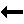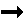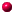What is this thing called Painlevé''?introductory examplesregular singular points Ordinary and Singular Points Ordinary differential equations (ODEs) come in many flavours. We will consider scalar ODEs governing a function y(x) of a complex variable x. A general such ODE looks like $$y^{(n)}=F(y^{(n-1)}, ..., y', y, x)\qquad (0.3)$$ We will concentrate on functions $$F$$ that are rational in $$y^{(n-1)}, ..., y', y$$. Moreover, we will assume that $$F$$ is analytic in $$x$$ except at a finite number of isolated points $$x_i$$, $$i=0, ..., s-1$$ where $$s$$ is a nonnegative integer. Definition: A point $$r$$ where $$F(y^{(n-1)}, ..., y', y, r)$$ is analytic is called an ordinary point of the ODE. The points $$x_0, x_1, ..., x_{s-1}$$ are called fixed singularities of the ODE. Equation (0.2) (from Section 0.0 ) is $$y'=- y^2/x\qquad\qquad (0.2)$$ Clearly $$x_0=0$$ is a fixed singularity. Excercise: Show that infinity is also a fixed singularity. (Change variables to $$t=1/x$$. Show that the resultant ODE for $$u(t)=y(1/x)$$ also has a fixed singularity at $$t=0$$.) However, any non-zero finite point $$x$$ is an ordinary point. The main theorem of existence and uniqueness for ODEs shows that every solution defined by a regular initial value problem given at an ordinary point must be analytic. For Equation (0.2), the general solution is $$y(x)=1/(\log(x)-a).$$ Define $$y(1)=1$$. Clearly the corresponding solution is $$y(x)=1/(\log(x)+1)$$ which is analytic at the initial point $$x=1$$, has a logarithmic singularity at $$x=0$$, and has a pole when $$\log(x)=-1$$, i.e., $$x=e^{-1}$$. If we change the initial condition to $$y(1)=-1$$, the corresponding solution becomes $$y(x)=1/(\log(x)-1)$$ This solution is analytic at the initial point $$x=1$$. It still has a logarithmic singularity at $$x=0$$, but its pole now occurs where $$\log(x)=+1$$, i.e., $$x=e^{1}$$. It is clear that the pole has moved to a different location.Back to ContentsNalini Joshi Last modified: 12 October 2011 by N.Joshi